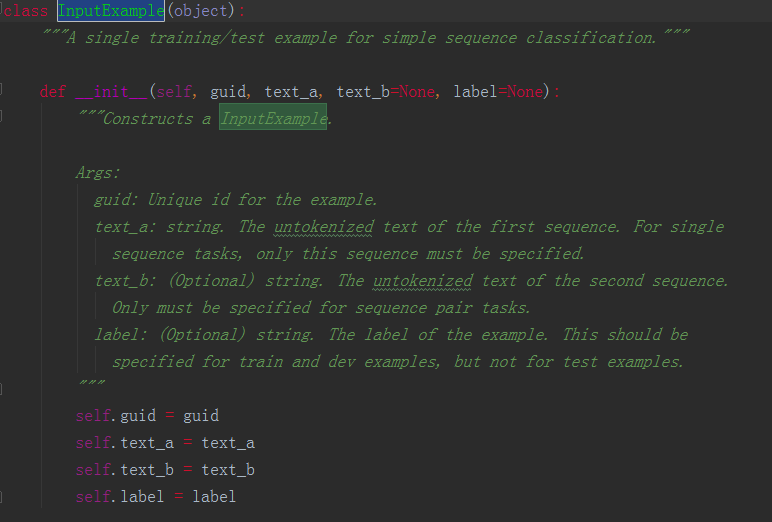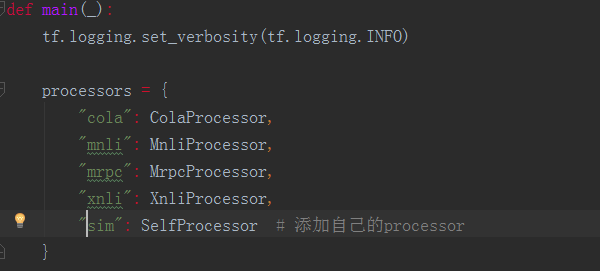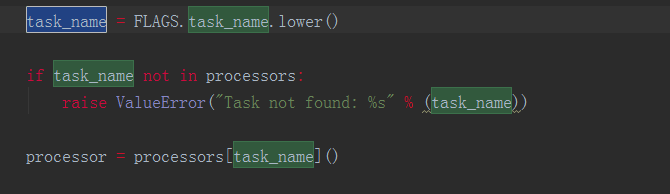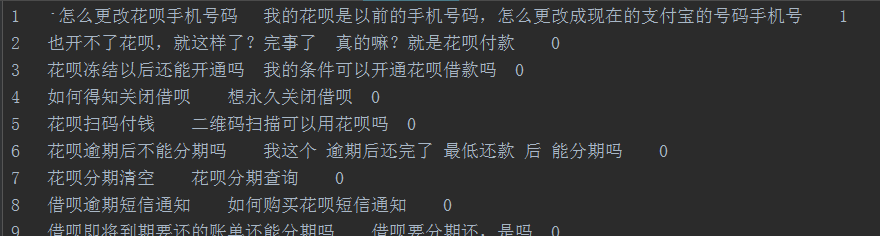BERT中文实战（文本相似度）

BERT本质上是一个两段式的NLP模型。第一个阶段叫做：Pre-training，跟WordEmbedding类似，利用现有无标记的语料训练一个语言模型。第二个阶段叫做：Fine-tuning，利用预训练好的语言模型，完成具体的NLP下游任务。

代码解析




data_dir指的是我们的输入数据的文件夹路径。查看代码，不难发现，作者给出了输入数据的格式：修改 processor

get_train_examples为例，函数需要返回一个由InputExample类组成的listInputExample类是一个很简单的类，只有初始化函数，需要传入的参数中guid是用来区分每个example的，可以按照train-%d'%(i)的方式进行定义。text_a是一串字符串，text_b则是另一串字符串。在进行后续输入处理后(BERT代码中已包含，不需要自己完成) text_a和text_b将组合成[CLS] text_a [SEP] text_b [SEP]的形式传入模型。最后一个参数label也是字符串的形式，label的内容需要保证出现在get_labels函数返回的list里。

class SelfProcessor(DataProcessor):
"""Processor for the CoLA data set (GLUE version)."""

def get_train_examples(self, data_dir):
file_path = os.path.join(data_dir, 'train.csv')
with open(file_path, 'r', encoding="utf-8") as f:
examples = []
guid = 'train-%d' % index
split_line = line.strip().split("\t")
print(split_line)
text_a = tokenization.convert_to_unicode(split_line)
text_b = tokenization.convert_to_unicode(split_line)
label = split_line
examples.append(InputExample(guid=guid, text_a=text_a,
text_b=text_b, label=label))
return examples

def get_dev_examples(self, data_dir):
file_path = os.path.join(data_dir, 'val.csv')
with open(file_path, 'r', encoding="utf-8") as f:
examples = []
guid = 'train-%d' % index
split_line = line.strip().split("\t")
text_a = tokenization.convert_to_unicode(split_line)
text_b = tokenization.convert_to_unicode(split_line)
label = split_line
examples.append(InputExample(guid=guid, text_a=text_a,
text_b=text_b, label=label))
return examples

def get_test_examples(self, data_dir):
"""See base class."""
file_path = os.path.join(data_dir, 'test.csv')
with open(file_path, 'r', encoding="utf-8") as f:
examples = []
guid = 'train-%d' % index
split_line = line.strip().split("\t")
text_a = tokenization.convert_to_unicode(split_line)
text_b = tokenization.convert_to_unicode(split_line)
label = split_line
examples.append(InputExample(guid=guid, text_a=text_a,
text_b=text_b, label=label))
return examples

def get_labels(self):
"""See base class."""
return ["0", "1"]

def _create_examples(self, lines, set_type):
"""Creates examples for the training and dev sets."""
examples = []
for (i, line) in enumerate(lines):
# Only the test set has a header
if set_type == "test" and i == 0:
continue
guid = "%s-%s" % (set_type, i)
if set_type == "test":
text_a = tokenization.convert_to_unicode(line)
label = "0"
else:
text_a = tokenization.convert_to_unicode(line)
label = tokenization.convert_to_unicode(line)
examples.append(
InputExample(guid=guid, text_a=text_a, text_b=None, label=label))
return examples运行 fine-tune

export BERT_BASE_DIR=/path/to/bert/chinese_L-12_H-768_A-12 #全局变量 下载的预训练bert地址
export MY_DATASET=/path/to/xnli #全局变量 数据集所在地址

python run_classifier.py
--do_train=true
--do_eval=true
--dopredict=true
--data_dir=$MY_DATASET 训练数据路径 --vocab_file=$BERT_BASE_DIR/vocab.txt
--bert_config_file=$BERT_BASE_DIR/bert_config.json --init_checkpoint=$BERT_BASE_DIR/bert_model.ckpt
--max_seq_length=128  #语句长度
--train_batch_size=32
--learning_rate=5e-5
--num_train_epochs=2.0
--output_dir=./sim_output/ #模型输出路径

BERT 源代码（其他）

BERT这次开源的代码，由于是考虑在google自己的TPU上高效地运行，因此采用的estimator是tf.contrib.tpu.TPUEstimator,虽然TPU的estimator同样可以在gpu和cpu上运行，但若想在gpu上更高效地做一些提升，可以考虑将其换成tf.estimator.Estimator,于此同时model_fn里一些tf.contrib.tpu.TPUEstimatorSpec也需要修改成tf.estimator.EstimatorSpec的形式，以及相关调用参数也需要做一些调整。在转换成较普通的estimator后便可以使用常用的方式对estimator进行处理，如生成用于部署的.pb文件等。